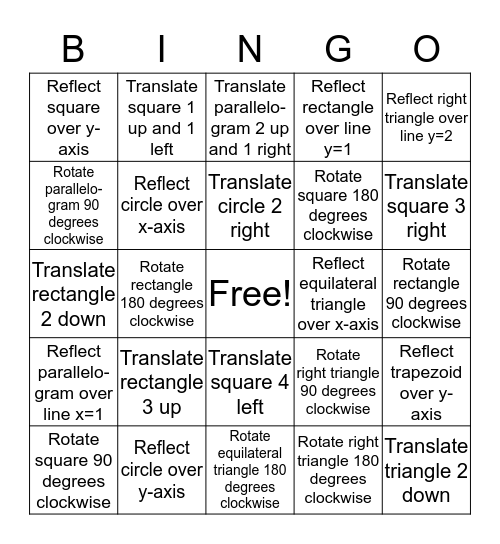# Transformation Bingo!This bingo card has a free space and 24 words: Translate rectangle 3 up, Reflect circle over y-axis, Translate circle 2 right, Rotate square 90 degrees clockwise, Reflect right triangle over line y=2, Reflect square over y-axis, Rotate parallelo-gram 90 degrees clockwise, Translate square 1 up and 1 left, Reflect equilateral triangle over x-axis, Translate square 4 left, Rotate square 180 degrees clockwise, Translate rectangle 2 down, Rotate right triangle 90 degrees clockwise, Reflect trapezoid over y-axis, Reflect circle over x-axis, Rotate right triangle 180 degrees clockwise, Rotate rectangle 90 degrees clockwise, Translate parallelo-gram 2 up and 1 right, Translate square 3 right, Rotate rectangle 180 degrees clockwise, Translate triangle 2 down, Reflect parallelo-gram over line x=1, Reflect rectangle over line y=1 and Rotate equilateral triangle 180 degrees clockwise.

## Play Online